Subsections

4. Learning Algorithm

THE FINAL CHAPTER OF THE PRELIMINARIES gives an overview of learning algorithms and how they can be used to mediate the semantic differences between different concurrency strategies. Given the context of open distributed systems, this problem cannot be solved automatically. This is because we cannot expect to have an adaptor available for every possible pair of conflicting interfaces, so we need some automatic approach.

We have a problem in which we need to create an adaptor that is able to establish meaningful communication between two components with a conflicting interface. As already mentioned in the introduction, the adaptor will be divided in three modules. One module, the liveness module, will be responsible for keeping the underlying component alive, a second module, the concurrency module, will be responsible for implementing a suitable concurrency strategy and a third module, the enforce-action module, is responsible for bypassing an existing concurrency strategy. The second and third modules can be implemented in a formal way, the first module however is computationally too complex to be able to handle formally (see liveness of Petri-nets in section 7.3.1). Therefore we will resort to a learning algorithm.

Before we can look for solutions to the problem presented we need to investigate which techniques we can use to keep the underlying component alive. We need to know what we can expect from a given learning algorithm and what kind of information it expects. In this chapter we first explain a number of important concerns with respect to learning algorithms in general, after which we will briefly introduce a number of different learning algorithms available. Secondly, based on this information we will explore the possibilities we have to map our problem on existing learning algorithms. Thirdly, we explain how genetic algorithms and genetic programs are conceived, and how we will use them in this dissertation. Fourthly, we present reinforcement learning algorithms.

4.1 A World of Variations

A LEARNING ALGORITHM IS CHARACTERIZED by the sort of problems presented, the sort of solutions to be found and the environment in which the algorithm is supposed to work. For instance, in our case we have a problem of conflicting interfaces: the solutions we are looking for are adaptors between the conflicting interfaces and the environment includes all the limitations we place upon the adaptors. Examples of such limitations are 'an adaptor is a process', or 'an adaptor can only handle one message at a time' to 'the conflicts we are dealing with are concurrency problems'. All these implicit or explicit degrees of freedom define the search space of the learning algorithm.

Sadly there is no universal learning algorithm that will work optimally in every possible situation. Therefore learning algorithms are designed to work within certain environments. Learning algorithms cannot learn what they cannot see, and they don't work very well if they see too much information. (This is the typical bias versus variance problem). This is a bit of a disappointment for people introduced to learning algorithms because they assume that an intelligent algorithm should be able to look for other clues than the ones found in their environment. Learning algorithms will not exhibit such behavior[Mor96]. Nevertheless they can be helpful in certain domains such as character recognition, robot motion planning, robot activator control, regulation systems and others.

The number of available learning algorithms is large, and the number of variations on every algorithm itself is even larger. We can consider different criteria for classifying learning algorithms. We will discuss them below:

• On-line/off-line learning: Some learning algorithms need to be trained off-line to learn how to react in certain situations. Once this is done the resulting configuration is fixed and used in an on-line situation. Consequently, when new problems occur the algorithm will not adapt itself further. Other algorithms on the other hand will immediately learn what to do in an on-line situation and doesn't require a separate off-line training phase.
• Supervised/non-supervised learning: supervised algorithms require categorized examples. After presenting these examples to the algorithm, adaptations are made to the configuration such that the different categories are recognized correctly in the future. With non-supervised learning, there is no explicit set of good and bad examples.
• Classifier/parameter tuning algorithms: Classifier algorithms are algorithms which learn to classify certain input into categories. On the other hand, some learning algorithms are designed to maintain a certain global requirement, which is influenced by a number of different interacting parameters. Parameter tuning algorithms are useful to find out the correct values for these parameters.
• Basic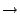general and generalbasic: Within the category of classifier algorithms two sub categories can be defined: basic to general learners and general to basic learners. The basic to general approach will try to find a suitable descriptive feature of a set of examples such that these examples belong to the same category. A general to basic approach initially places all examples in the same category, and afterward tries to split large categories into more specific, smaller sub-categories.
• Numerical/symbolic learning: certain learning algorithms work with integer or float values to represent the environment, while others work with symbols (discrete values) to do so. Numerical approaches are very well suited in regulation systems.
• State/stateless: some learning algorithms are stateless in the sense that they cannot remember previous events. Other algorithms do have state and can, when necessary, recall a previous event.
• The biologically inspired model they simulate: these can be genetic algorithms, neural networks, reinforcement learning or others.
Because we have a large number of criteria for categorizing learning algorithms, in practice selection of a suitable algorithm is a very complex process. Somewhere, there is an abstract notion of a learning algorithm (a neural network, a genetic algorithm, a classifier system) which is made very specific, depending on the situation in which it should work. Therefore we will investigate the applicability of a number of learning algorithms by tuning certain aspects of the algorithm. However, before doing so, we present a number of different learning strategies:

• Tree Searches: Breadth-first, depth-first, priority-first searches: by searching the entire solution space we are almost guaranteed to find a the best solution. The environment is presented in an abstract form, the possible actions are enumerated and the possible outcomes are defined. By checking every possible solution the algorithm can finally come up with the best answer. The problem here is that most often, the search space is too large to be able to find anything useful within a reasonable amount of time. Nevertheless, this technique is often used: chess-programs with a minimum-maximum strategy are an example. Usually, programs relying on this kind of problem-solving introduce some kind of pruning: a method of weeding out complete branches that are assumed not to contain useful solutions. However, using pruning is a dangerous and very delicate technique that requires excellent domain knowledge.
• Genetic Algorithms/Programming: Genetic algorithms, which are inspired on a model of evolution as defined by Darwin [Dar59] (the survival of the fittest) and Mendel [Gre65] (properties of sexual reproduction). The idea behind genetic algorithms is that a population of solutions is measured in a certain environment. The test assigns a fitness to every individual. An individual that is not fit enough dies and is replaced by a child of 2 individuals with a high fitness. This way (and by means of mutation), new individuals are created and the overall fitness of the population rises. Genetic programs are genetic algorithms in which the individuals are programs and fitness is measured by executing the programs.
• Reinforcement learning [SAG98] is an on-line technique. In contrast to the above methods, reinforcement learning algorithms are defined by the kind of problems they solves. A reinforcement learning problem is a situation in which a learner needs to learn which actions should be taken to maximize the received reward in the long run. Typically there is an interaction between the actions, the environment and the rewards: after taking some action, a, possibly delayed, reward is assigned and the environment will probably change. Based on the reward (or punishment) the learner receives, its behavior will be altered in the future. A typical problem of this approach is the tradeoff between exploring the environment and exploiting its knowledge.

4.2 Mapping our Problem onto a Learning Algorithm

TO CHOSE A SUITABLE LEARNING ALGORITHM for our problem, we need to define the boundaries clearly.

• On-line/off-line: Whether the adaptor should be trained on-line or off-line is an important question because of the nature of open distributed systems: the behavior of components may change at any time. We could argue that training an adaptor off-line in a real world setting, with the ability to reset participating actors, and afterward inserting it in the running system would produce a suitable result. However, this is not the case because most often an off-line trained adaptor behaves statically when inserted in the running system: it will not adapt to previously unencountered behavior. This means that when such a situation occurs the adaptor is completely at a loss and won't work properly. Therefore we need an on-line learner. However, when we use an on-line algorithm we should be absolutely sure that the algorithm doesn't place participating components in an unstable state. Hence it should only try out things that are allowed in the given context.
• Supervised/non-supervised: The algorithm should work in a non-supervised way, simply because we cannot define a suitable supervisor in an open distributed system. Furthermore supervised learning is usually performed off-line, which we have chosen not to do. For the sake of the argument, assuming that we could use an off-line learning strategy, then still it would be very difficult to give specific examples of good or bad concurrency strategies. We will come back to this issue in chapter 7.
• Symbolic/numerical: a characteristic of our case is that the environment is, to a large extent, symbolic: the actions presented at an interface are discrete. The difference between a JoinActor message and an IsFree message is as important as the difference between an IsFree message and an Unlock message. Therefore we need a learning algorithm which works on symbolic input and symbolic output. Processing the environmental input can be either symbolic or numerical. However, because the nature of the interface adaptors is typically a problem of interaction conversion: 'when to send what', processing the input is preferably symbolic.
Given these characteristics of our problem, reinforcement learning seems to be a correct choice to match our requirements. However, other approaches such as priority-first searches and genetic algorithms will become very useful to investigate the power of certain representations. Therefore, in the sections below we will introduce genetic algorithms and discuss the basics of a reinforcement learning technique.

4.3 Genetic Algorithms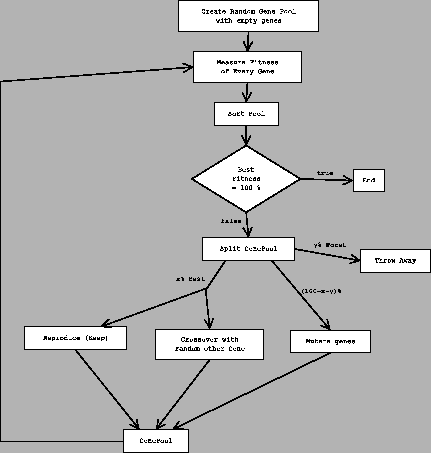BELOW WE EXPLAIN THE DETAILS OF A GENETIC ALGORITHM. We will also explain how we use them as representational testers in this dissertation.

A genetic algorithm [Gol89] is an algorithm that tries to solve a problem by trying out a number of possible solutions, called individuals. Every individual is an encoding of a number of modifiable parameters, called genes, and is assigned a fitness that measures how well the individual solves the problem at hand. From the pool of individuals a new generation of individuals is created. This can be either by preserving, mutating or crossing over individuals. This process is repeated until a suitable individual is found. An illustrative flowchart of this process is pictured in figure 4.1.

The standard questions before implementing any genetic algorithm are: What are the individuals and their genes? How do we represent the individuals? How do we define and measure the fitness of an individual? How do we initially create individuals? How do we mutate them and how do we create a cross-over of two individuals? How do we compute a new generation from an existing one? At the moment a genetic algorithm is implemented in a way that the individuals are themselves programs, we call it a genetic programming algorithm. In our implementation, the individuals could be protocol adaptors between communicating processes.

4.3.1 Genetic Algorithms as Representational Testers

Genetic algorithms as an evolutionary algorithm to solve the problem of conflicting concurrency strategies is relatively useless in a running system, because a genetic algorithm works in general off-line and can never guarantee that the resulting adaptor will work in every possible situation. Indeed: it is very difficult to verify certain concurrency requirements such as deadlock-freedom or no race conditions. However, we will use genetic algorithms is such a way that we turn an argument against them to our advantage. This argument is the representational problem: how well does a genetic algorithm perform given a certain representation of the problem [SB92,Sch87]. Or, stated otherwise how can we create a representation that will offer a good fitness landscape.

To illustrate this, think of a problem in which an ant has to learn how to walk a line from left to right. If we use a representation of our individuals in which we only offer the generation process 'go left' and 'go right' primitives, the genetic algorithm will very easily find a solution to the problem. However if we use another representation of the individuals with operators such as 'current angle', 'current position', 'turn left x degrees', 'turn right x degrees', 'forward' and 'backward' it will be substantially more difficult to find a solution to the same problem. We will use this property of genetic algorithms (which is, in general, a big disadvantage), to determine the best representation of a solution within a given environment and fitness function. Specifically, we will keep the same environment and fitness function and will measure how fast a solution is found given a certain representation of the individuals. We consider that if a certain problem can be solved easily under a certain representation, it is a good representation. If the same genetic algorithm has more trouble finding a solution the representation might not be so suitable. In chapter 9 and chapter 11 we will use this technique to validate different representations.

However, which kind of representation are available for our problem still needs to be discussed. In general two approaches are used. First, we can use hierarchically structured, human readable/programmable programming languages such as Scheme, Lisp, Java and others to represent the behavior of the concurrency adaptor. Unfortunately, the inevitable syntactic structure imposed by these languages complicates the random generation of programs. Second, we can use a representation that is more suitable for random generation, but is in general not readable. These approaches are mainly based on classifier systems, for which we will explain the basics below.

4.3.2 Classifier Systems

We will now introduce one of the representations we have tested because we need them in chapter 11. This representation are classifier systems. Classifier systems [Gol89] in cooperation with genetic algorithms form a genetic programming4.1 approach that is symbolic and easy to implement. Moreover, given our problem, their symbolic nature can be to our advantage.

A classifier system is a kind of control system that has an input interface, a finite message list, a classifier list and an output interface. The input and output interfaces put and get messages to and from the classifier list. The classifier list takes a message list as input and produces a new message list as output. Every message in the message list is a fixed-length binary string that is matched against a set of classifier rules. A classifier rule contains a number of (possibly negated) conditions and an action. These conditions and actions form the genes of each individual in our genetic algorithm. Conditions and actions are both ternary strings (of 0, 1 and #). #' is a pass-through character that, in a condition, means either 0 or 1 matches'. If found in an action, we simply replace it with the character from the original message. Table 4.1 shows a very simple example. When evaluating a classifier system, all rules are checked (possibly in parallel) with all available input messages. The result of every classifier evaluation is added to the end result. This result is the output message list. For more details, we refer to [Gol89].

Table 4.1: Illustration of how actions produce a result when the conditions match all messages in the input message list. ~ is negation of the next condition. A disjunction of two conditions is used for each classifier rule. The second rule does not match for input message 001. The third rule does not match because the negated condition is not satisfied for input message 001.
Input message list = { 001, 101, 110, 100 }

 Condition Action Matches Result 00#    101

Output message list = { 111, 100, 110 }

Using this representation for individuals of a genetic algorithm, we can easily introduce the necessary operators: cross-over is performed by selecting certain bits within the classifier expressions (genes) and exchanging them with the same genes from another individual. Mutation is easily implemented by selecting a number of bits and simply changing them to something else. The technique of using entire classifier systems as individuals within a genetic program is referred to as the Pittsburgh approach [Smi]. In comparison, the Michigan approach [LF93] treats every classifier- rule as an individual. After having introduced genetic algorithms and classifier systems we will now proceed with discussing reinforcement learning algorithms.

4.4 Reinforcement learning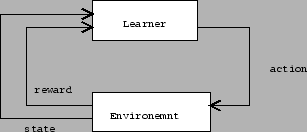THE APPROACH WE WILL USE to learn how to keep Petri-nets alive will be based on a reinforcement learning technique. Therefore, we will now introduce what a reinforcement learning technique is. Reinforcement learning [SAG98,KLAPM96] is originally an on-line technique where a learner tries to maximize the accumulated reward it receives from the environment. The actions the learner can take in a given situation are initially unknown, so the learner itself needs to discover a good way of working. The typical challenge for a reinforcement learning algorithm is that actions taken in a certain context will influence the future environment and rewards the learner will get.

Typical to the class of reinforcement learning algorithms is that it is defined based on the problem at hand. Reinforcement learning is defined as a solution to the problem in which a learner, which interacts with its environment, tries to reach a certain goal. Such a learner should be emerged in the environment, it should be able to sense the environment and it should be able to act upon the environment. The goal, or goals of such a problem should also be related to and expressible in the environment. A more formal definition of the problem in a more formal way will be given in section 4.4.4.

There are many kinds of reinforcement learning algorithms. For this dissertation, we will focus on one particular branch of algorithms, called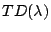-learners [Sut88,Tes92,SAG98]. The following sections will discuss this branch of algorithms in more detail. We will first introduce the elements most often encountered in reinforcement learners, second we will state the difference between episodic and continual tasks. Third, we will formally express the requirements of a reinforcement learning problem by means of Markov Decision Processes. Fourth, we will explore the value function and policy of a-learner. Fifth we will discuss the tradeoff between exploration and exploitation.

4.4.1 A Typical Reinforcement Learner

A typical reinforcement learning algorithm contains 4 elements:

1. A policy, which defines the actions the learner will take in a given context. This is typically what should be learned.
2. A reward function which defines the goal(s) of the learner as a numerical value. This function defines the immediate reward the learner gets from the environment. For instance, a learner searching for food gets a reward when it perceives food at its sensors.
3. A value function, which defines the actions that are good in the long run. Generally speaking, the value of a certain situation is the maximum reward the learner can expect to receive starting from that situation.
4. A model of the environment which represents what actions will turn one situation into another. In the original reinforcement approaches, models were avoided and only trial-and-error search was possible. However, some recent reinforcement learning algorithm approaches benefit from the use of models.

In general, a reinforcement learning algorithm works on-line, i.e. emerged in the environment, without supervision. There is never an explicit training phase that teaches how the algorithm should behave in a real environment. However sometimes the same learner will be allowed multiple trials, in the form of episodes, to perform a task. An episode is the run of a learner until a terminal state is reached. Such a terminal state can be either a dead-state or a goal-state. After reaching such a state, a new episode starts, in which the same exact problem as before needs to be solved. However, during every episode the learner will be able to develop its behavior further until a suitable approach has been reached. Such a task is called an episodic task.

Other learning-tasks don't have terminal states and simply require the learner to maximize the total amount of reward it receives in the long run. Such a task is called a continual task.

4.4.3 Elements of a Reinforcement Learning Problem

Given the above general description of a reinforcement learning problem, we can now formally introduce the elements of a reinforcement learning algorithm. In chapter 9, we will use this formal approach to map the liveness problem to a reinforcement learning problem.

The action a learner takes at time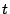will be called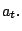The actions available to the learner in a situation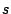are denoted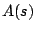. When an action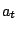is executed the resulting situation is denoted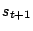. The reward received after executingis a numerical valueTime is considered to be discrete.

4.4.4 Markov Decision Processes

A reinforcement learner can never take all possible information into account when deciding upon its action, because either a) not all information is available, or b) it would require too much space to store all information. Therefore reinforcement learners require the environment to offer just enough information for the learner such that it can proceed. The situation-signal a learner receives should summarize in a compact way the past which has lead to this situation, such that no relevant information has been lost. If the state-signal succeeds in retaining this information it is said to have the Markov-property. For example, a checkers position, containing the position of all pieces on board, has the Markov-property, because it summarizes the past in a way such that no necessary information is lost for the future.

Formally, if the situation-signal has the Markov-property, the next situation and reward can be probabilistically expressed in terms of the current situation and reward. In the equation below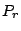denotes a probability distribution.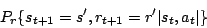This notation denotes a chance that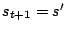and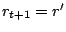if both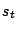andare given. In summary, a problem needs to have the Markov property before it can be considered to be a reinforcement learning problem.

4.4.5 The Value Function & Policy

We will now describe the value functions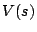,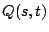and policy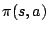as used by many reinforcement learners. A policy describes which action is favored in a given situation. To decide this, the policy uses a value-function, which describes the maximum possible reward a certain action could lead to. Typically, both the policy and the value function change over time. The policy balances the exploration and exploitation phases while the value function learns how good it is to be in a certain state.

Formally, given a certain situation, the policy decides the probability of an action to be chosen: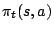returns the probability that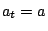if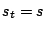. The value function defines what the maximum future reward will be given a certain policy. However, because the time a learner might run can be infinite, the expected future reward may be also be infinite. Therefore, a discount factor is introduced which makes future rewards less interesting if they are placed further in the future.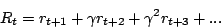(4.1)

where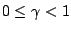.

Given this definition of a future reward, we can define the state-value function, which defines how good it is for a learner to be in a certain state. Of course, this depends on which actions the learner will take in certain situations, hence the policy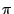should be taken into account.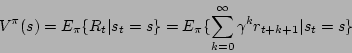In the above equation,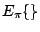denotes the expected return value given that the learner follows policy. Similarly, we can define the expected return the learner receives when it takes action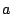in state.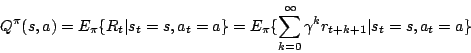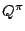is called the action-value function under policy.

Both the state-value as action-value functions cannot be calculated immediately because the expected future reward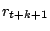is unknown. The goal of most reinforcement learning algorithms is to find approximations of these value-functions. In chapter 9 we will investigate how we can map the liveness problem (and our solution) to a function approximation of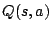.

• One such a technique estimates these functions by keeping track of all mean rewards following a certain state under a certain policy. This kind of estimation methods are called Monte Carlo methods. This technique however requires the existence of episodes, otherwise different action-decisions cannot be measured and the necessary averages of future rewards cannot be calculated. [AdFDJ03]
• If a perfect model of the environment is present, the value-functions are known and as such techniques such as dynamic programming can be used. However, they are computationally very heavy and most often there is no perfect model of the environment. [KLAPM96]
• Other estimation methods such as Q-learning [PW94] do a function approximation of the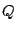value-function. The technique used by this methods is called temporal difference learning because rewards are backwards propagated and over time the correct future rewards will be known. We will describe this technique in detail in section 9.3.2.

4.4.6 Exploration versus Exploitation

The difference between the policy and the value-function is that a value-function estimates what future reward could be possible, while the policy decides which action will effectively be taken. However, this action is not always necessarily the action that will lead to the highest reward. If the best action is taken, the policy is exploiting the value-function, otherwise it is exploring the environment. A tradeoff between exploitation and exploration must be made. If a learner exploits too much it will be blind to possibly better solutions which could result in higher rewards, while a learner which keeps on exploring the environment in a fully random way will end up accumulating very little reward.

An action selection method in which all information is exploited without ever trying new paths is called a greedy action selection strategy. The drawback of such a method is clearly that it gets easy stuck in sub-optimal solutions. A simple but effective variant of greedy action selection methods are the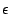-action selection methods, in which most of the time the best available action is favored, but in% of the time an action is uniformly random selected from the set of possible actions. Eventually, all possible actions will be selected once by this method.

This wraps up our introduction of reinforcement learning.

4.5 Summary

IN THIS SECTION we have explained that we need an on-line adaptive learning algorithm that works on symbolic input and output. The algorithm needs to work on-line because of the nature of open distributed systems. The algorithm needs to work with symbolic input and output because of the nature of interfaces. The internal representation however can be either numerical or symbolic. As a result the only algorithm suitable to do the job is reinforcement learning, for which we explained the basic operation.

Second, in this chapter we introduced the use of genetic algorithms as a means to test the representation of a system, instead of an approach to solve a problem. If a certain problem can be solved easily under a certain representation, it is a good representation. If the same genetic algorithm has more trouble finding a solution the representation might not be so suitable. On the same representational track we also introduced classifier systems because we will investigate their usefulness chapter 11.

Third, we introduced the working of reinforcement learning algorithms. We explained the policy-function, value-function, reward function and the requirements posed upon a problem before it can be considered to be a reinforcement learning problem. One of these requirements was that the environment signal should summarize the past in such a way that no necessary information is lost. This property is called Markov. In chapter 9 we will need this property to ensure that the approach we use is indeed a valid reinforcement learning problem. The reinforcement learning technique introduced in this chapter will be used to create the liveness module.

We have now presented the preliminaries of this dissertation. In chapter 2 we explained the event based system we will use. In chapter 3 we introduced the use of Petri-nets as a means to formally specify an interface and in chapter 4 we introduced the learning algorithms we will need later on. Given these preliminaries we are now able to shift our attention to the case we will use to validate our thesis: concurrency strategy conflicts in open distributed systems.

Footnotes

... programming4.1
On the other hand, if we use classifier systems in a Michigan approach, then, strictly speaking it is not genetic programming anymore.
Werner 2004-03-07